# K-Means ++

## Introduction

K-Means, an unsupervised machine learning algorithm, used to group similar data points into similar clusters. This blog will focus mainly on K-means++, the extended version of the K-means algorithm. It would be best if you visited Understanding K-means to get the idea of its extension.

## Limitations of K-means

Let's discuss the drawback of the K-means clustering algorithm with an example.

Suppose we have n data points in multidimensional space. For simplicity, let's assume it is in a 2-D plane.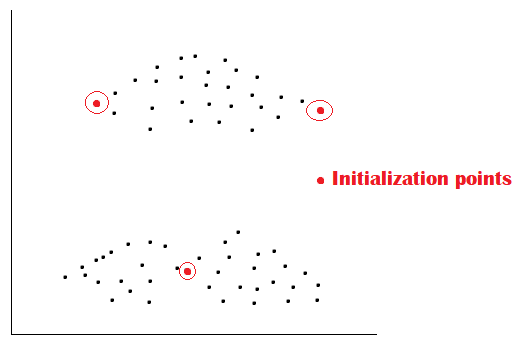If we run K-means algorithms on these data points, the initial step would be to randomly select K ( K=3 in this case) points as the centroids. Suppose the initialization points are those marked in red. Then, the clusters formed will look like this.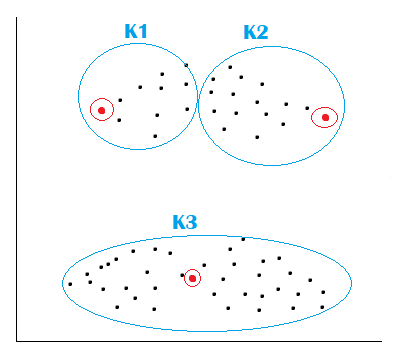If the initialization points were different in the above case, the clusters formed would look like this.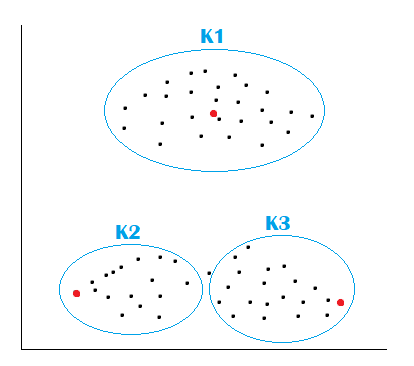## K-means++

Different initialization points lead to other clusters; this is the problem with the K-means. So we have to choose a method to initialize the centroids better. The concept of K-means++ is introduced, which is used for initialization. The algorithm says that;

1. Randomly select the first Centroid.
2. For each data point, calculate the distance to the nearest Centroid.
3. Select the next Centroid. The probability of choosing the next Centroid is directly proportional to the distance.
4. Then Repeat steps 2 and 3 for K centroids.

Here the distance is Euclidean or Manhattan distance.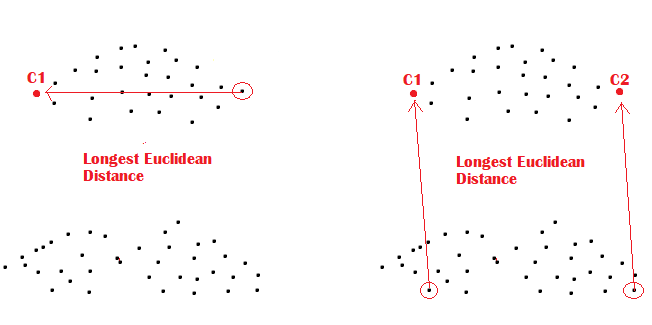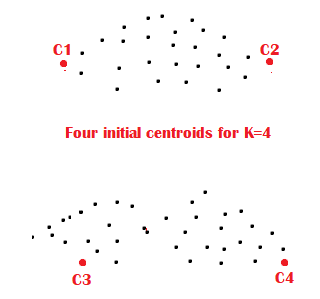In this way, K-means++ chooses K initial centroids. After selecting the centroids, we can run K-means algorithms on the datasets.

Q1) What is the Euclidean distance used in the K-means algorithm?

=>Euclidean distance is the distance measured between pairs of points in n-dimensional space. If there are n coordinates, then the distance between two points p and q is given by-

Euclidean distance = i=1n(pi-qi)2

Q2) What is the Manhattan distance used in the K-means algorithm?

=> Manhattan distance between two points p and q in space is their pairwise absolute difference. If there are n coordinates, then the distance between two points p and q is given by-

i=1n|pi-qi|

Q3) How do we calculate the centroid in the K-means algorithm?

=> For n data points, i th coordinate of centroid is given by

xi = 1npi , where p is individual data points.

Q4) What is the major issue in the implementation of K-means++?

=> K-means++ is computationally expensive to implement as compared to K-means. The run-time for convergence to optimum is drastically reduced for K-means++.

Q5) How does Outliers affect the K-means algorithm?

=>Outlier will cause the Centroid to be displaced from its actual position, or the Outlier might get their clusters instead of being ignored. So we should remove Outliers before clustering.

## Key Takeaways

We come to the end of the discussion. There are various exciting clustering algorithms in Unsupervised learning. You can learn them in great detail with hands-on projects from- Link.

Don’t forget to check: Unsupervised Learning.

THANK YOU